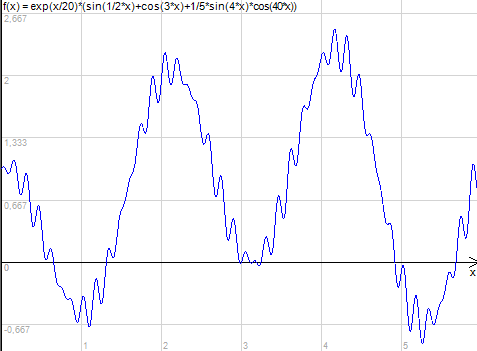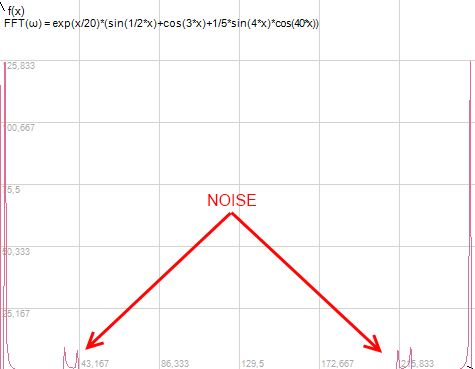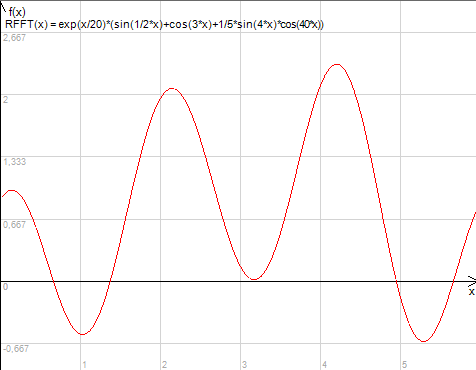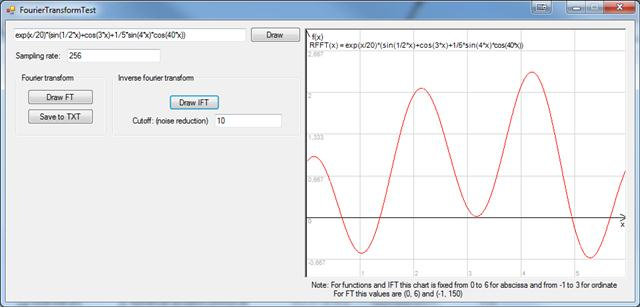### Features And Algorithms

This library uses numerical calculator library so can handle same functions at input.

Mathematical formula used in this library is normal variant of Fourier Transform (not the Fast Fourier Transform).

### Example

Take function f(x)=exp(x/20)*(sin(1/2*x)+cos(3*x)+1/5*sin(4*x)*cos(40*x))

Graph of this function shows it's not smooth:You can use Fourier Transform to reduce noise and improve function smoothness. Fourier Transform (abscissa from 0 to 6, 256 samples) of this function look like this:You can clearly see two groups of noisy frequencies. Lets reset all values less then 10 to 0 (reduce noise) and then invert the transformation. Our function now look like this:### Usage

Note: PointC is class similar to Point that additionally contains imaginary element. It comes with NumericalCalculatorCommon.dll which is inside this library compressed archive.

`string function = "exp(x/20)*(sin(1/2*x)+cos(3*x)+1/5*sin(4*x)*cos(40*x))";int sampling = 256;double abscissaFrom = 0d;double abscissaTo = 6d;double cutoff = 10d;List<PointC> pointsFT = new List<PointC>();List<PointC> denoisedFunctionPoints = new List<PointC>();//Computing FTFourierTransform ft = new FourierTransform();pointsFT = ft.Compute(function, sampling, abscissaFrom, abscissaTo);//Cutofffor (int i = 0; i < pointsFT.Count; i++){    PointC pc = pointsFT[i];    if (Math.Abs(pc.Y.Real) < cutoff)        pc.Y = 0;    pointsFT[i] = pc;}//Inverse FTdenoisedFunctionPoints = ft.ComputeInverse(pointsFT, sampling, abscissaFrom, abscissaTo);`

### Example application